• Размер: 583.5 Кб
• Количество слайдов: 19

## Описание презентации Important Information : • Steve is по слайдам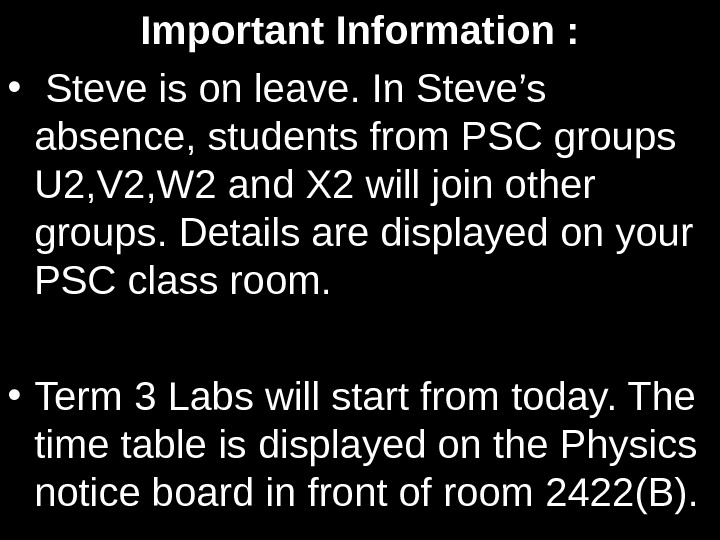Important Information : • Steve is on leave. In Steve’s absence, students from PSC groups U 2, V 2, W 2 and X 2 will join other groups. Details are displayed on your PSC class room. • Term 3 Labs will start from today. The time table is displayed on the Physics notice board in front of room 2422(B).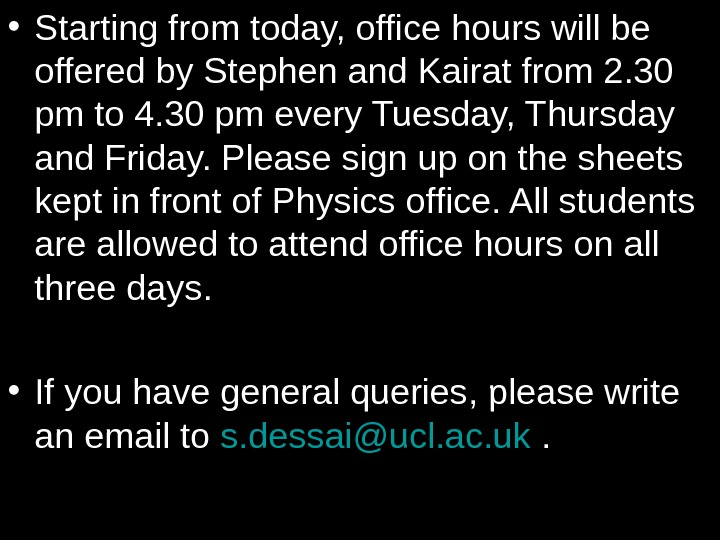• Starting from today, office hours will be offered by Stephen and Kairat from 2. 30 pm to 4. 30 pm every Tuesday, Thursday and Friday. Please sign up on the sheets kept in front of Physics office. All students are allowed to attend office hours on all three days. • If you have general queries, please write an email to s. [email protected]cl. ac. uk .At the end of this lecture you should: • Know what DC electricity is • Have an understanding of mean free path and drift velocity in a conductor and be able to derive an expression for the latter • Have a qualitative understanding of how conductors and semiconductors carry electric charge • Know what Ohms law is and how to draw basic characteristics for different conductors • Be able to define resistance , the volt and electromotive force • Be able to define resistivity and conductivity and perform calculations which demonstrate your understanding • Be able to derive a simple expression for electrical power. L 33 — DC Electricity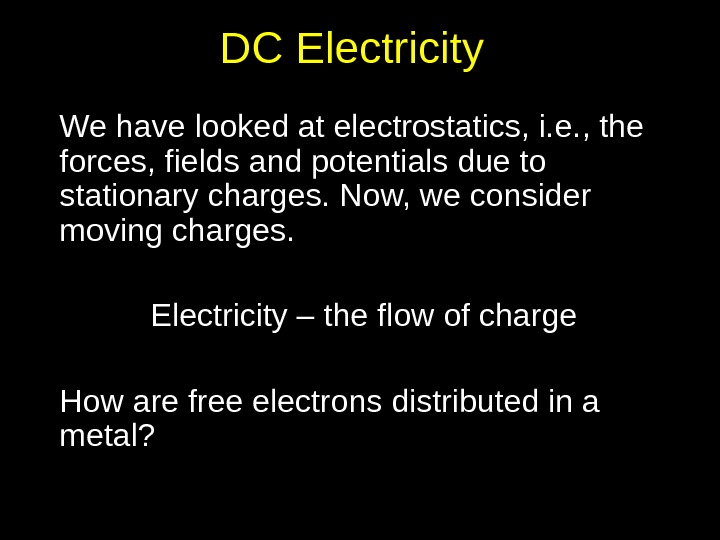DC Electricity We have looked at electrostatics, i. e. , the forces, fields and potentials due to stationary charges. Now, we consider moving charges. Electricity – the flow of charge How are free electrons distributed in a metal?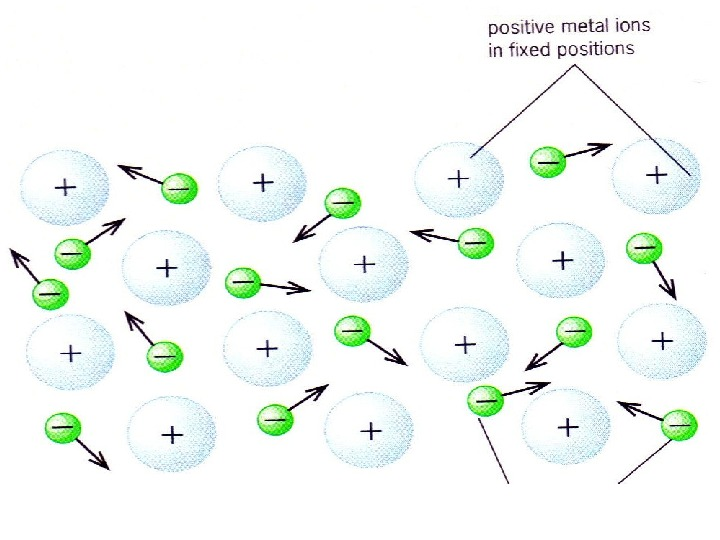Conduction in solids • In a conductor, e. g. copper, every atom has a ‘free’ electron. If copper is in its solid state, these electrons move randomly at high speed (about c/1000 m s -1 ). • In this sea of electrons, no net flow of charge. • However, if a battery is connected, an electric field is created…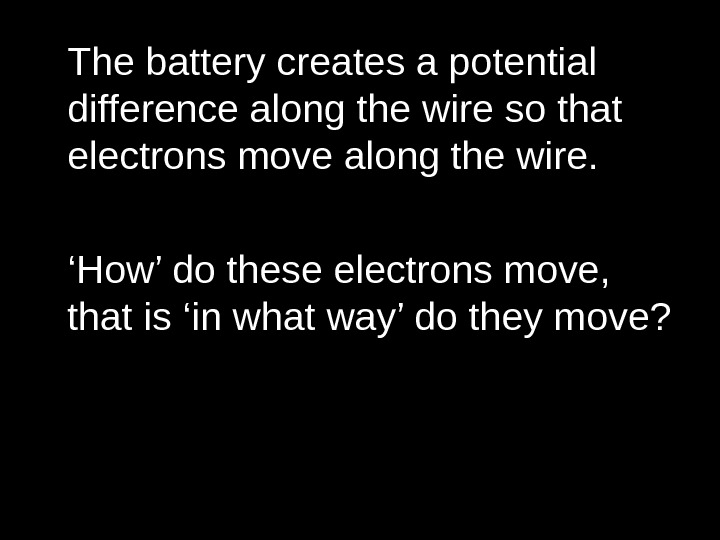The battery creates a potential difference along the wire so that electrons move along the wire. ‘ How’ do these electrons move, that is ‘in what way’ do they move?Average drift velocity — derivation Consider charge carriers that pass area A per unit time. Let number of charge carriers per unit volume be n.Insulators and semiconductors • Some materials have no free electrons; e. g. , crystals such as diamond and glass, and plastics. These insulators do not permit a flow of charge. • Other materials have very few free electrons. In semiconducting materials e. g. , silicon (sand), electrons must have higher drift velocity to provide current compared to a metal.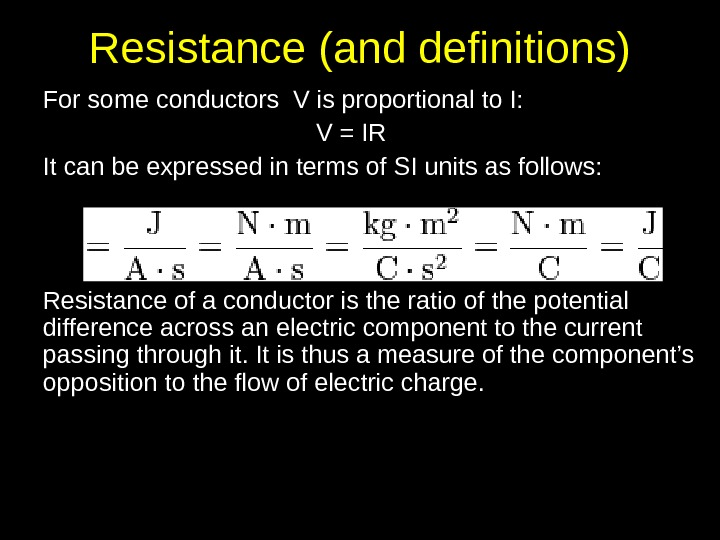Resistance (and definitions) For some conductors V is proportional to I: V = IR It can be expressed in terms of SI units as follows: Resistance of a conductor is the ratio of the potential difference across an electric component to the current passing through it. It is thus a measure of the component’s opposition to the flow of electric charge.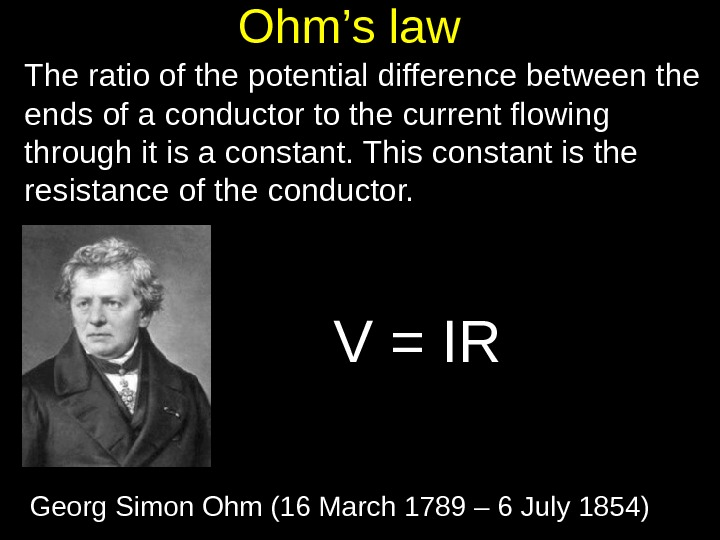Ohm’s law The ratio of the potential difference between the ends of a conductor to the current flowing through it is a constant. This constant is the resistance of the conductor. V = IR Georg Simon Ohm (16 March 1789 – 6 July 1854)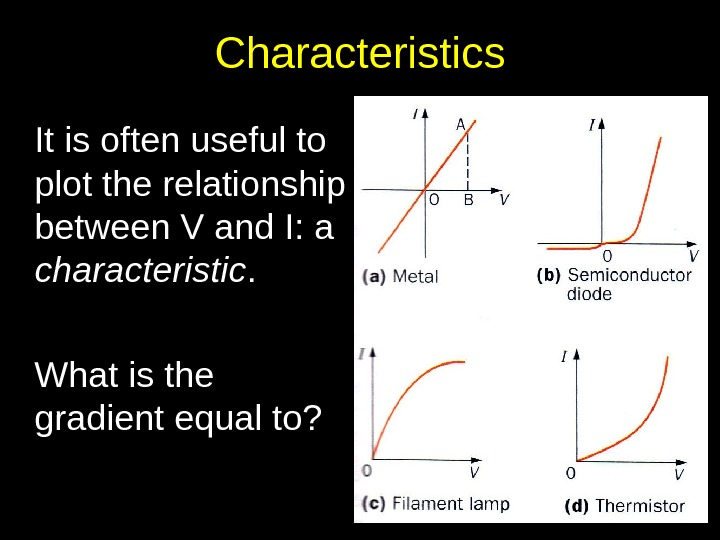Characteristics It is often useful to plot the relationship between V and I: a characteristic. What is the gradient equal to?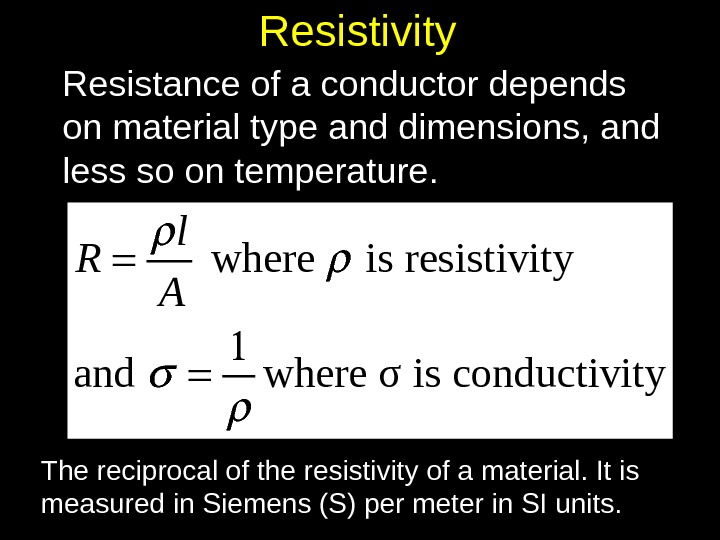Resistivity Resistance of a conductor depends on material type and dimensions, and less so on temperature. where is resistivity and where σ is conductivity l R A The reciprocal of the resistivity of a material. It is measured in Siemens (S) per meter in SI units.e. m. f. (electromotive force) The e. m. f. is NOT a force. It is a measure of the strength of a battery and has units volts or J C-1. amount of energy converted into electrical energy e. m. f. = charge passed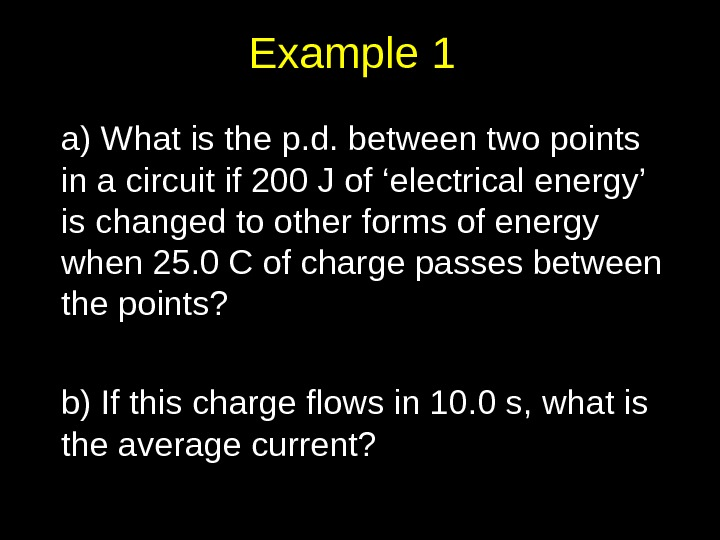Example 1 a) What is the p. d. between two points in a circuit if 200 J of ‘electrical energy’ is changed to other forms of energy when 25. 0 C of charge passes between the points? b) If this charge flows in 10. 0 s, what is the average current?Example 2 a) Copper’s density is 8930 kg m -3. Assuming each copper atom has one free electron, find the number of free electrons per cubic metre. b) A copper wire is 25. 0 m long and has a cross-sectional area of 1. 00 x 10 -6 m 2. What is the total free charge, in C, in the wire? c) Assuming these electrons are responsible for the electric current, how long will it take this charge to travel 25. 0 m when 2. 00 A flows? d) What is the drift velocity of these free electrons? (Note: Atomic mass of Cu is 63. 546 g/mol)Example 3 Prove that the electrical power delivered when a charge Δ Q moves across a p. d. Δ V in a time Δ t is given by P = I Δ V.Example 4 The density of a copper wire is three times that of an aluminium wire, and the resistivity of copper is half that of aluminium. Find the ratio of the masses of copper and aluminium wires of equal resistance and length.Checklist • Adams and Allday: 4. 1, 4. 2, 4. 3, 4. 7, 4. 8, 4. 9. • Serway: 17. 1 – 17. 5, 17. 8 • At the end of this lecture you should: • Know what DC electricity is • Have an understanding of mean free path and drift velocity in a conductor and be able to derive an expression for the latter • Have a qualitative understanding of how conductors and semiconductors carry electric charge • Know what Ohms law is and how to draw basic characteristics for different conductors • Be able to define resistance , the volt and electromotive force • Be able to define resistivity and conductivity and perform calculations which demonstrate your understanding • Be able to derive a simple expression for electrical power